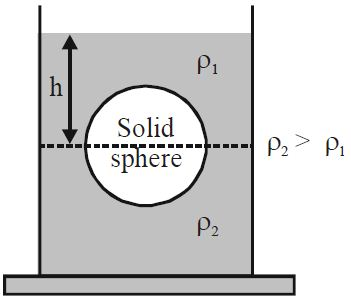# Buoyancy + Force +Pressure!A solid sphere of radius $r$ is floating at the interface of two immiscible liquids of densities $\rho_1$ and $\rho_2$ where $\rho_2 >\rho_1$, half of its volume lying in each zone. The height of the upper liquid column from the interface of the two liquids is $h$.

Find the force exerted on the sphere by contact with the upper liquid layer.

Details

• atmospheric pressure = $p_0$
• acceleration due to gravity is $g$
×

Problem Loading...

Note Loading...

Set Loading...